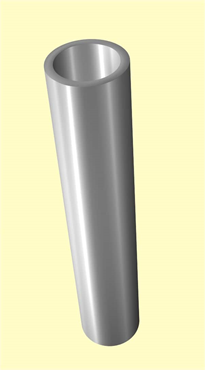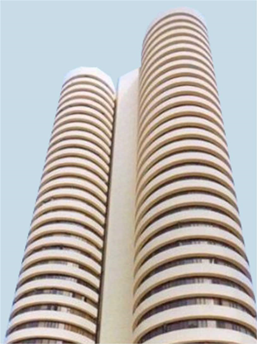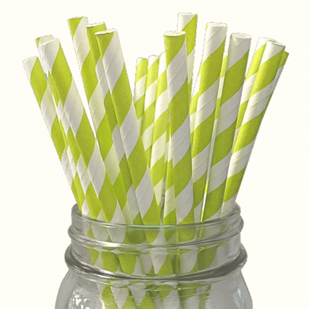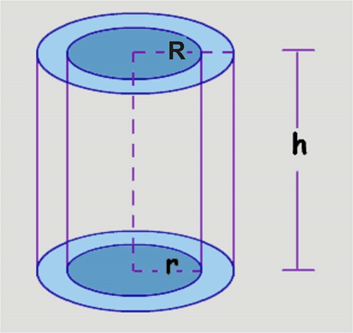Area of Hollow Cylinder

Parts of a Cylinder - Curved and Total Surface Area of a Hollow Cylinder

A solid surface produced by a line moving parallel to a fixed line, while its end describes a closed figure in a plane is called a cylinder. A cylinder is the limiting case of a prism. This old-fashioned view is utilized in fundamental utilizations of geometry, yet the unpredictable numerical perspective has moved to the endless curvilinear surface and this is the manner by which a cylinder is currently all around characterized in a few present-day parts of geometry and topology. The shift in the straightforward meaning has created some uncertainty with terminology.

If a line is perpendicular to the base, the cylinder is called a Right cylinder, otherwise, it is called an oblique cylinder. The line joining the centers of the bases is called the axis of the cylinder. A hollow cylinder is a cylinder which is vacant from inside and has some difference between the internal and external radius.

Parts of a cylinder:

Base and side

A cylinder is a solid that is common to see in regular day to day existence, for example, a straw. In the event that you dismantle it, you discover it has two closures, called bases, that are normally roundabout. The bases are consistent and parallel to one another. If you somehow managed to 'unroll' the cylinder you would locate the side is really a rectangle shape when straightened out.

Height

The height h is the perpendicular distance between the 2 bases. It is important to use the perpendicular height ('altitude') when we calculate the volume of a slanted cylinder.

The radius r of a cylinder is the radius of the base. If you are given the diameter instead, remember to take half of it.

Axis

A line joining the center of each of the 2 bases.

Real Life examples:

1. Tubes2. Circular Buildings3. StrawsDeducing the formulae of areas of a hollow cylinder:If R is the outer radius of the cylinder and r is the inner radius of the cylinder, then
(i) Volume (the solid portion) = Volume of external cylinder-volume of internal cylinder
= π R2 h -π r2 h
= π (R2- r2) h

(ii) Lateral surface area = External surface area of a cylinder + Internal surface area of a cylinder
= 2πRh + 2πrh
=2πh(R + r)

(iii) Total surface area = Lateral surface area + Areas of solid bases
=2πh(R + r) + 2π(R2- r2)

Example:

Find the weight, lateral surface area and total surface area of a steel pipe whose interior and exterior diameters measure 15cm and 17cm respectively, and length 10m; one cubic cm of iron weighing 0.8gm.

Solution:

Here d = 15cm r = 7.5cm
D = 17cm R = 8.5cm
h = 10m = 1000cm

Volume = π(R2-r2 )h
= π(72.25 – 56.25)1000
= 50265.48 cm3.

Weight = Volume x density = 50265.48 x 0.8 = 40212.39 gms

Lateral surface area = 2π(R + r)h
= 2π(8.5 + 7.5)1000
= 2π x 16 x 1000
= 100530.96 cm2.

Total surface area of the pipe = Lateral surface area of pipe + Area of bases
=100530.96 + 100.53
= 100631.49 cm2.

Example:

A hollow cylinder copper pipe is 21dm long. Its outer diameter and inner diameter are 10cm and 6cm respectively. Find the volume of copper used in manufacturing the pipe.

Solution:

Given that:

The height of the cylindrical pipe is h = 21dm = 210cm
Thus, External radius, R = 10/2 = 5cm
Internal radius, r = 6/2 = 3cm
The volume of the copper used in manufacturing the pipe
= Volume of external cylinder − volume of an internal cylinder
= πR2h − πr2h
= π(R2−r2)h
= 22/7 [52−32]×210 = 22/7 × 16 × 210
= 22×16×30
= 10560 cu.cm

Example:

The inner radius of a circular well is 2.1 m and its depth is 21 m. Find the cost of plastering the inner surface of a circular well at the rate of Rs. 40 per m2

Solution:

Given: Radius of the circular well (r) = 2.1 m, depth (h) = 21 m

Here we need to plaster the inner surface of the well which is the sum of Curved surface area and Area of the base.

Area to be plastered = Curved surface area + Area of base
= 2 πrh + πr2
= 2 x 22/7 x 2.1 x 21 + 22/7 x (2.1)2
= 277.2 + 13.86
= 291.06 m2

Cost of 1 m2 of well = Rs. 40
Cost of 291.06 m2of well = Rs. (40 × 291.06) = Rs. 11642.40

Test Sample:

Example:

The lateral surface area of a hollow tube is 4224 cm2. Later on it has been cut along its height and formed a rectangular sheet of width 33 cm. Find the perimeter of a rectangular sheet?

Example:

The total surface area of a hollow metal tube, open at both ends of external radius 8 cm and height 10 cm is 338π cm2. Taking r to be the inner radius, provide an equation in r and use it to state the thickness of the metal in the cylinder.

Example:

The total surface area of a hollow ceramic cylinder which is open from both the sides is 4620 sq. cm, the base ring area is 115.5 sq. cm and height 7 cm. Find the thickness of the ceramic cylinder.